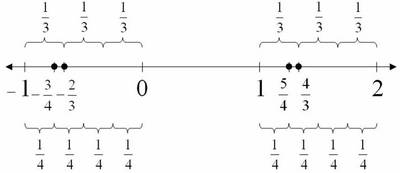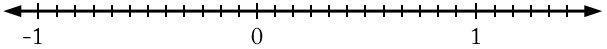# Fractions on the Number Line

Alignments to Content Standards: 6.NS.C.7.a1. Find and label the numbers $\frac43$, $\frac 54$, $-\frac23$, and $-\frac34$ on the number line.

2. For each of the following, state which inequality is true. Use the number line diagram to help explain your answers.

1. Is $\frac43 \gt \frac54$, or is $\frac43 \lt \frac54$?

2. Is $-\frac23\gt -\frac34$, or is $-\frac23 \lt -\frac34$?

3. Is $-\frac34$ closer to $0$ or is $\frac54$? Explain how you know.

## Solutions

Solution: Reasoning about the position relative to $1$ or $-1$

This solution depends on two insights:

• seeing the position of the fractions relative to $1$ or $-1$
• seeing that thirds are bigger than fourths.
1. Mark the points -1,0,1,2 at regular intervals on a number line. For the positive fractions, notice that $\frac{5}{4}$ is $\frac{1}{4}$ more than $1 = \frac44$ and that $\frac{4}{3}$ is $\frac13$ more than $1 =\frac{3}{3}$. Choose a point one-fourth of the way between $1$ and $2$, label it $\frac{5}{4}$. To the right of that choose the point one-third of the way between $1$ and $2$ and mark the point $\frac{4}{3}$. Similarly, $-\frac{3}{4}$ is $\frac14$ more than $-1$ and $-\frac{2}{3}$ is $\frac13$ more than $-1$. Choose a point one-fourth of the way between $-1$ and $0$, label it $-\frac{3}{4}$. A little to the right of it is the point $\frac13$ of the way between $-1$ and $0$; mark it $-\frac{2}{3}$.2. Note: On a number line where positive numbers are to the right of zero and negative numbers are to the left of zero, numbers farther to the right are always greater than those to the left.

1. $\frac43 > \frac54$

$\frac43$ is to the right of $\frac54$, so $\frac43$ is greater than $\frac54$.

2. $-\frac23 > -\frac34$

$-\frac23$ is to the right of $-\frac34$, so $-\frac23$ is greater than $-\frac34$.

3. $-\frac34$ is closer to 0 than $\frac54$. $-\frac34$ is three unit fractions to the left of 0, while $\frac54$ is five of the same unit fraction to the right of 0.

Solution: Using a common denominator

Another way to plot all of these numbers on the same number line is to first find a common denominator.

A common multiple of 3 and 4 is 12, so we can use 12 as a common denominator of $\frac43$,$\frac54$,$-\frac23$ and $-\frac34$ twelve.

$$\frac43=\frac{16}{12} \qquad \frac54=\frac{15}{12} \qquad -\frac23=-\frac{8}{12} \qquad -\frac34=-\frac{9}{12}$$

Now we can add more hash marks to our number line in increments of $\frac{1}{12}$.Finally we can plot the numbers given.The remaining two parts of the question can be answered in a similar way to the first solution.